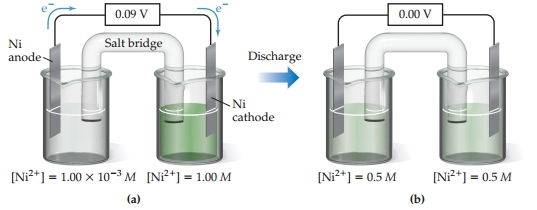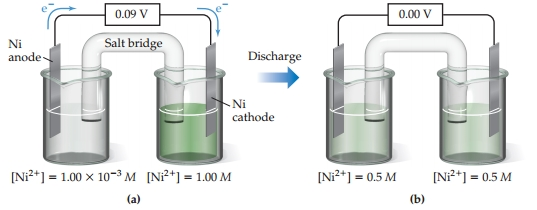# Problem: Concentration cell based on the Ni2+-Ni cell reaction. (a) Concentrations of Ni2+(aq) in the two half-cells are unequal, and the cell generates an electrical current and a voltage. (b) The cell operates until [Ni2+] is the same in the two half-cells, at which point the cell has reached equilibrium and the emf goes to zero.Which electrode, if any, loses mass as the reaction proceeds?

###### FREE Expert Solution

We’re being asked to determine which electrode loses mass as the reaction proceeds. We’re given the following diagram:Recall that a voltaic or galvanic cell is an electrochemical cell that is spontaneous and produces electricity

Let's recall some parts of the galvanic cell:###### Problem DetailsConcentration cell based on the Ni2+-Ni cell reaction. (a) Concentrations of Ni2+(aq) in the two half-cells are unequal, and the cell generates an electrical current and a voltage. (b) The cell operates until [Ni2+] is the same in the two half-cells, at which point the cell has reached equilibrium and the emf goes to zero.

Which electrode, if any, loses mass as the reaction proceeds?